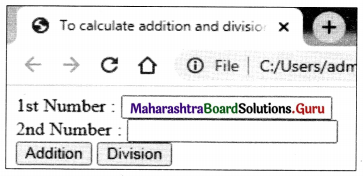# Maharashtra Board Class 11 Information Technology Practicals Skill Set 3 Client Side Scripting (JavaScript)

Balbharati Maharashtra State Board Class 11 Information Technology Solutions Practicals Skill Set 3 Client Side Scripting (JavaScript) Textbook Exercise Questions and Answers.

## Maharashtra State Board Class 11 Information Technology Practicals Skill Set 3 Client Side Scripting (JavaScript)

SOP 1: Create a JavaScript program for the following using appropriate variables, JavaScript inbuilt functions, and control structures.

• To accept an integer and display the result by multiplying it with 3.
• To accept two integers and display a larger number of them.
• To check whether the user entered number is positive or negative.

To accept integer and display the result by multiplying it with 3.
<!DOCTYPE html>
<title>To accept integer and display the result by multiplying it with 3.</title>
<body>
<script language=”javascript”> var a,no,ans;
a=prompt(Enter any value’);
no=parseInt(a);
ans=no*3;
document.
</script>
</body>
</html>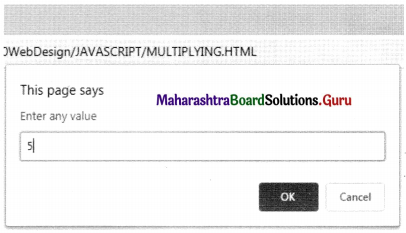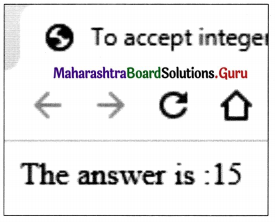To accept two integers and display larger number of them.
<!DOCTYPE html>
<title>To accept two integers and display larger number of them.</title>
<body>
<script language=”javascript”>
var a,b;
a=prompt(‘Enter first value’);
b=prompt(‘Enter second value’);
if(a>b)
document.write(“a is large number than b “);
else
document. write(“b is large number than a”);
</script>
</body>
</html>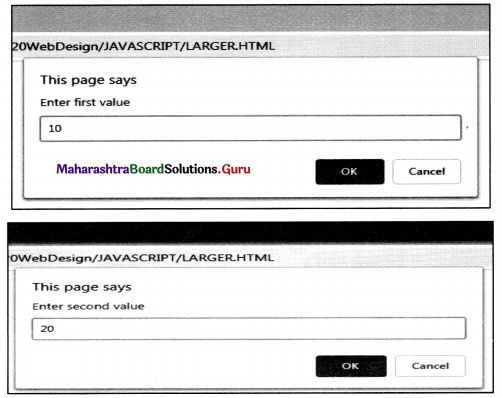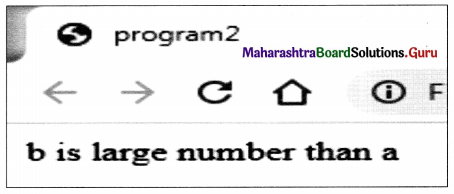To check whether, user entered number is positive or negative.
<!DOCTYPE html>
<title>To check whether, user entered number is positive or negative</title>
<body>
<script language=”javascript”>
var a,no;
a=prompt(‘Enter any number’);
no=parseInt(a);
if(no>0)
document.write(“Number is Positive”);
else
document.write(“Number is Negative”);
</script>
</body>
</html>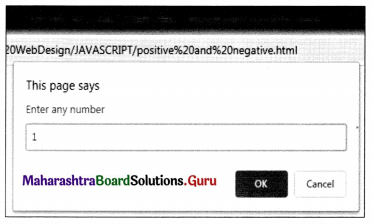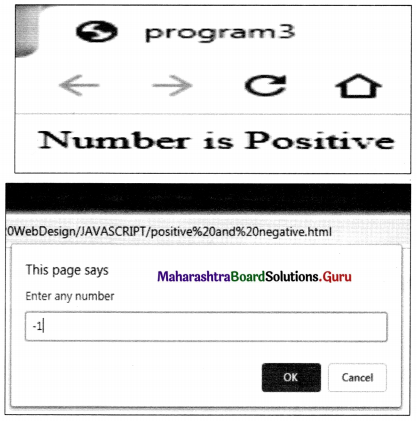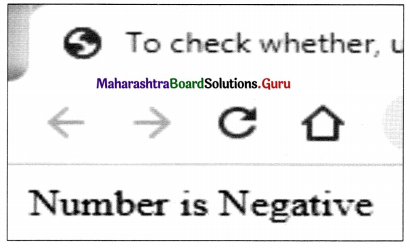SOP 2: Create a JavaScript program for the following using appropriate variables, JavaScript inbuilt functions, and control structures.

• To accept two positive or negative numbers and check whether they are equal or not.
• To accept a number and display the square of it.
• To check whether the accepted integer is multiple of 3 or multiple of 7.

To accept two positive or negative numbers and check whether they are equal or not.
<!DOCTYPE html>
<title>program3</title>
<body>
<script language=”javascript”> var no1,no2;
no1=prompt(‘Enter first number’);
no2=prompt(‘Enter Second number’);
if(no1==no2)
document.write(“Both are equal”);
else
document.write(“Given numbers are not equal”);
</script>
</body>
</html>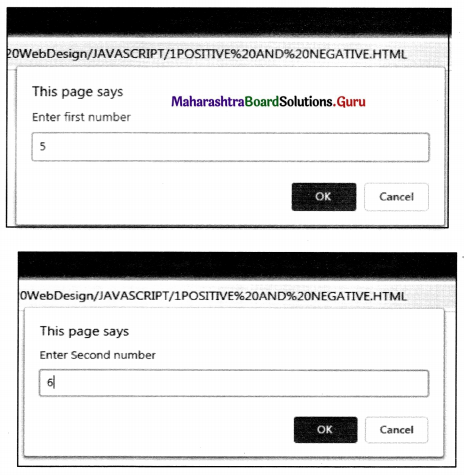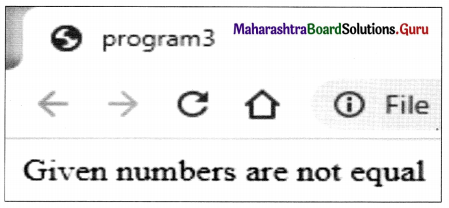To accept number and display square of it.
<!DOCTYPE html>
<title>To accept number and display square of it</title>
<body>
<script language-’j avascript”>
var no,sqr;
no=prompt(‘Enter Any number’);
sqr=no*no;
document, write (“The Square is=”+sqr);
</script>
</body>
</html>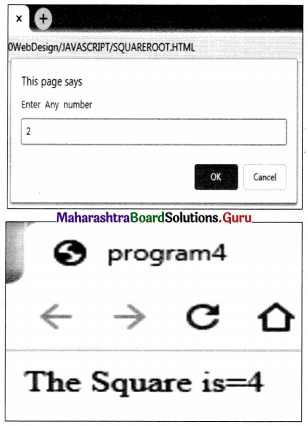To check whether the accepted integer is multiple of 3 or multiple of 7.
<!DOCTYPE html>
<html>
<title>To check whether the accepted integer is multiple of 3 or multiple of 7.</title>
<body>
<script language=JavaScript>
var a;
a=prompt(“Enter your first interger / number”);
if(a%3==0 | | a%7==0)
else
alert(“not a multiple of 3 or 7”);
</script>
</body>
</html>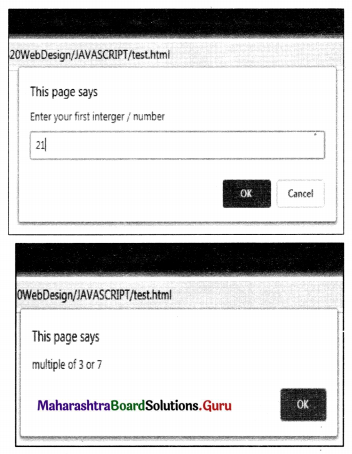SOP 3: Create a JavaScript program for the following using appropriate variables, JavaScript inbuilt string functions, and control structures.

• To accept a string and calculate its length.
• To accept a string and display it in lowercase and uppercase.
• To check whether the length of the string is 4 or greater.

To accept a string and calculate its length.
<!DOCTYPE html>
<title>program8</title>
<body>
<script language=”javascript”>
var a;
a=prompt(‘Enter string’);
document.write(“The length is=”+a.length);
</script>
</body>
</html>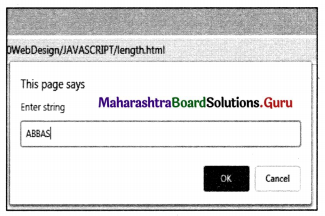To accept string and display it into lowercase and uppercase.
<!DOCTYPE html>
<title> To accept string and display it into lowercase and uppercase</title>
<body>
<script language=”javascript”>
var a;
a=prompt(‘Enter any string’);
document.write(“<br>Entering Strings “+a);
document.write(“<br>Lowercase=”+a.toLowerCase());
document.writeln(“<br>Uppercase=”+a.toUpperCase());
</script>
</body>
</html>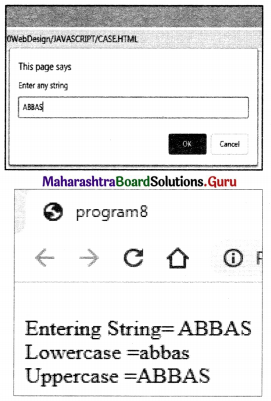To check whether the length of string is 4 or greater.
<!DOCTYPE html>
<html>
<title> JavaScript</title>
<body>
<script language=JavaScript>
var a,b;
b=a.length;
if(b=4 || b>=4)
else
</script>
</body>
</html>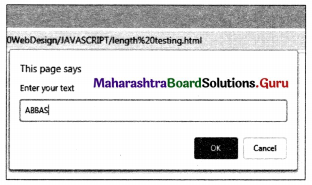SOP 4: Create event-driven JavaScript programs for the following using appropriate variables, JavaScript inbuilt functions, and control structures.
To accept numbers and validate if the given value is a number or not by clicking on the button.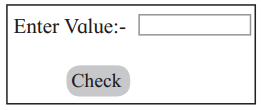To calculate the addition and division of two numbers.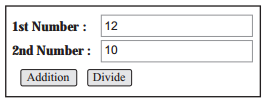To accept number and validate if the given value is a number or not by click
<!DOCTYPE html>
<html>
<title> To accept number and validate if the given value is a number or not by clicking on the button.
</title>
<script language-’JavaScript”>
function display()
{
var a,b;
a=form1.t1;value;
if(a>=0)
else
}
</script>
</body>
<form name=form1>
Enter Value: <Input type=text name=t1><br><br>
<Input type=button value=Check onClick=”display()”>
</form>
</body>
</html>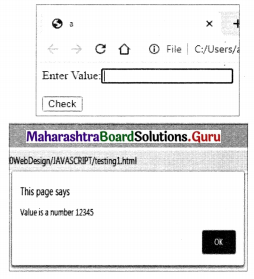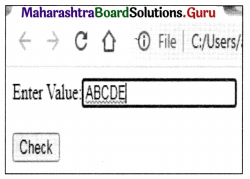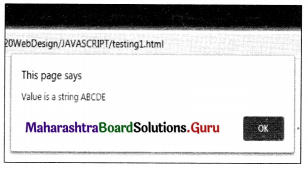To calculate addition and division of two numbers.
<!DOCTYPE html>
<title>To calculate addition and division of two numbers.</title>
<script langauge=”javascript”>
{
var a,b,result;
a=f1.t1.value;
b=f1.t2.value;
result=parselnt(a)+parselnt(b);
}
function div()
{
var a,b,d;
a=f1.t1.value;
b=f1.t2.value;
d=parselnt(a)/parselnt(b);
document.write(“The Divide is =”+d);
}
</script>